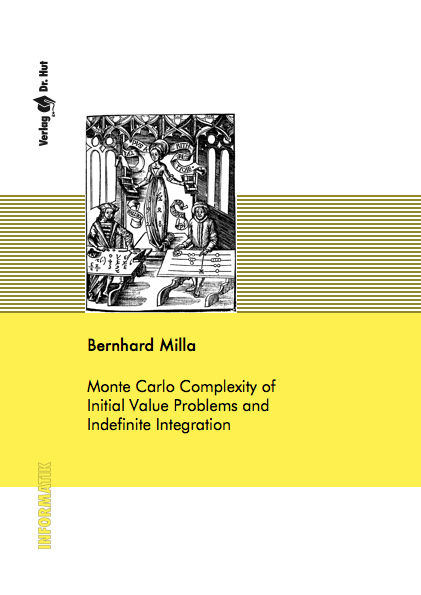Datenbestand vom 13. Juni 2019WICHTIGER HINWEIS
DER VERLAG IST IN DER ZEIT VOM 12.06.2019 BIS 23.06.2019 AUSCHLIESSLICH PER EMAIL ERREICHBAR.

aktualisiert am 13. Juni 2019

ISBN 9783843901697Euro 60,00 inkl. 7% MwSt

978-3-8439-0169-7, Reihe Informatik

Bernhard Milla
Monte Carlo Complexity of Initial Value Problems and Indefinite Integration

110 Seiten, Dissertation Technische Universität Kaiserslautern (2011), Softcover, A5

Zusammenfassung / Abstract

This thesis consists of two major parts. In the first one we study the complexity of randomized solution of initial value problems for systems of ordinary differential equations. We assume the input functions to be γ-smooth, where γ = r + ρ, which means that the r-th derivatives satisfy a ρ-Hölder condition.

In the second major part we study the problem of indefinite integration for functions f ∈ Lp([0, 1]d). We show that for 1 ≤ p ≤ ∞, the family of integrals [0,x] f(t)dt (x = (x1,...,xd) ∈ [0,1]d) can be approximated by a randomized algorithm uniformly over x ∈ [0, 1]d with the same rate n−1+1/ min(p,2) as the optimal rate for a single integral, where n is the number of samples. We present two algorithms, one being of optimal order, the other up to logarithmic factors. We also prove lower bounds and discuss the dependence of the constants in the error estimates on the dimension.